Скачать презентацию Estimating Continuing Value FIN 591 Financial Fundamentals Valuation 1

fbcbdb35a6b6f04fc6b7f9fb1964c320.ppt

• Количество слайдов: 18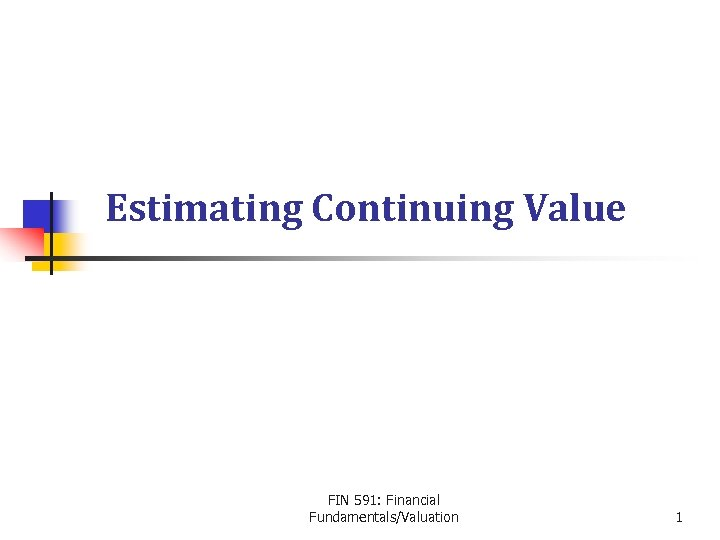Estimating Continuing Value FIN 591: Financial Fundamentals/Valuation 1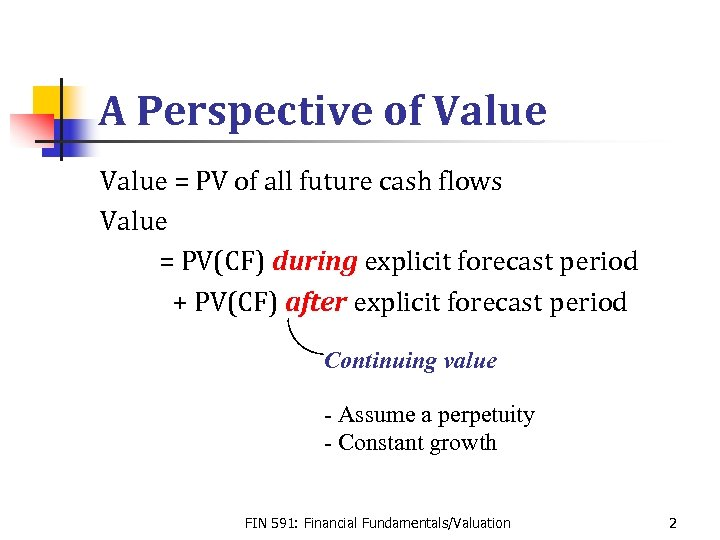A Perspective of Value = PV of all future cash flows Value = PV(CF) during explicit forecast period + PV(CF) after explicit forecast period Continuing value - Assume a perpetuity - Constant growth FIN 591: Financial Fundamentals/Valuation 2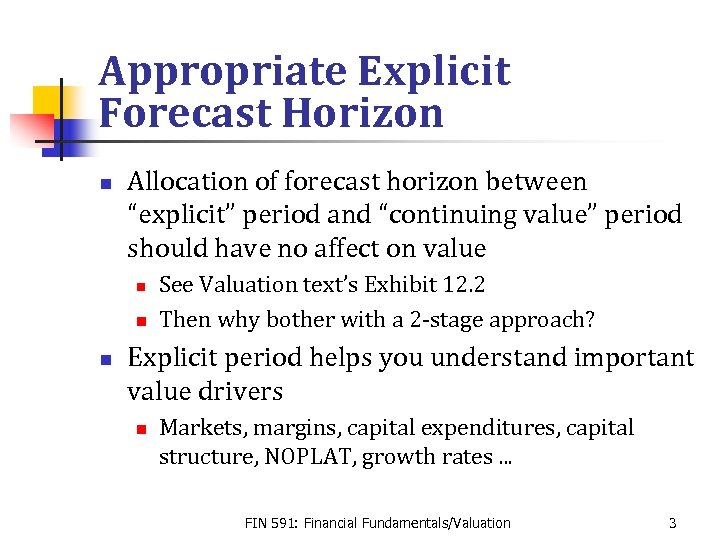Appropriate Explicit Forecast Horizon n Allocation of forecast horizon between “explicit” period and “continuing value” period should have no affect on value n n n See Valuation text’s Exhibit 12. 2 Then why bother with a 2 -stage approach? Explicit period helps you understand important value drivers n Markets, margins, capital expenditures, capital structure, NOPLAT, growth rates. . . FIN 591: Financial Fundamentals/Valuation 3Discounted Cash Flow n n Long explicit forecast Growing free cash flow perpetuity formula Value-driver formula If underlying assumptions of consistent n Three methods give the same continuing value. FIN 591: Financial Fundamentals/Valuation 4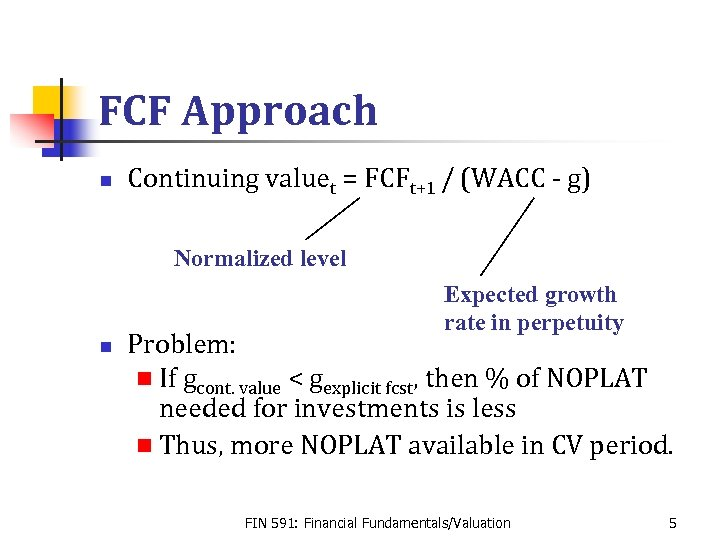FCF Approach n Continuing valuet = FCFt+1 / (WACC - g) Normalized level Expected growth rate in perpetuity n Problem: n If gcont. value < gexplicit fcst, then % of NOPLAT needed for investments is less n Thus, more NOPLAT available in CV period. FIN 591: Financial Fundamentals/Valuation 5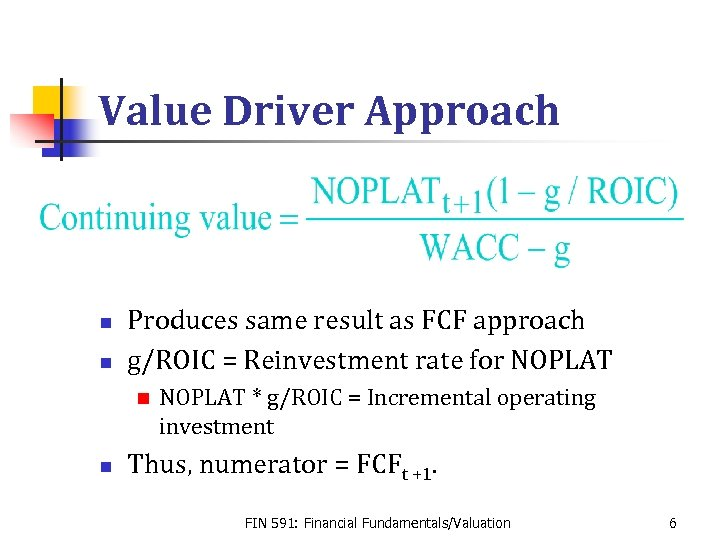Value Driver Approach n n Produces same result as FCF approach g/ROIC = Reinvestment rate for NOPLAT n n NOPLAT * g/ROIC = Incremental operating investment Thus, numerator = FCFt +1. FIN 591: Financial Fundamentals/Valuation 6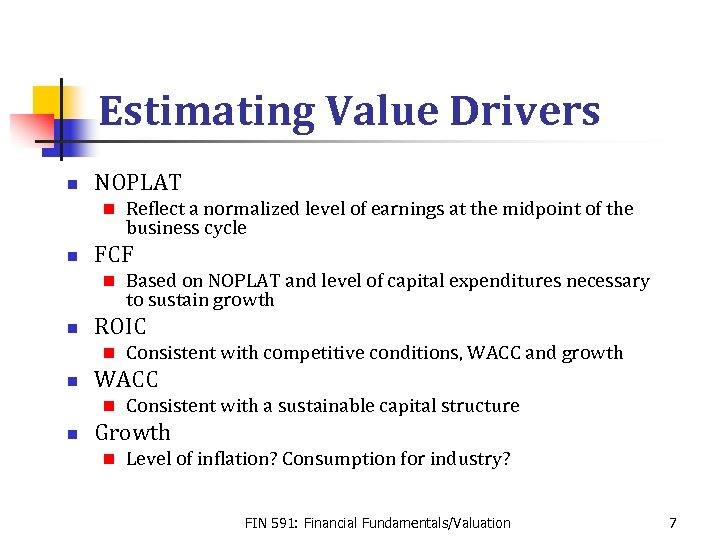Estimating Value Drivers n NOPLAT n n FCF n n Consistent with competitive conditions, WACC and growth WACC n n Based on NOPLAT and level of capital expenditures necessary to sustain growth ROIC n n Reflect a normalized level of earnings at the midpoint of the business cycle Consistent with a sustainable capital structure Growth n Level of inflation? Consumption for industry? FIN 591: Financial Fundamentals/Valuation 7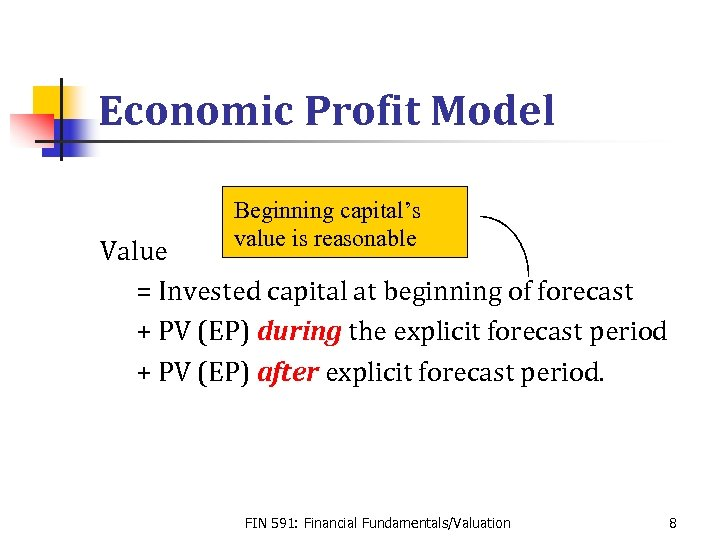Economic Profit Model Beginning capital’s value is reasonable Value = Invested capital at beginning of forecast + PV (EP) during the explicit forecast period + PV (EP) after explicit forecast period. FIN 591: Financial Fundamentals/Valuation 8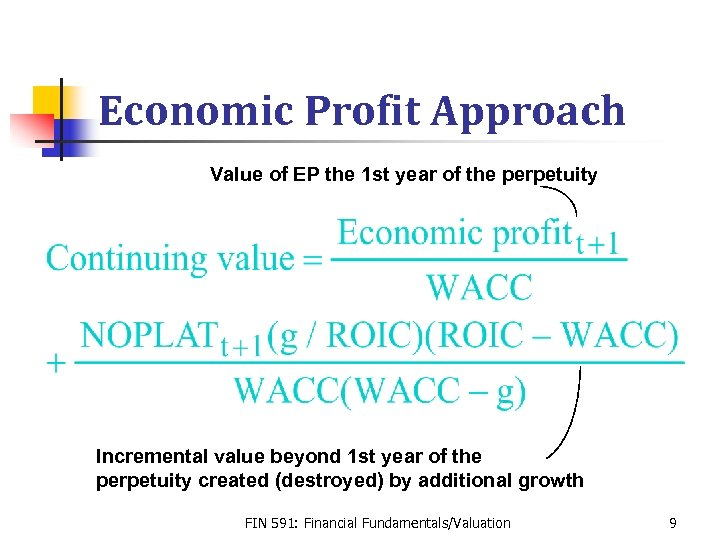Economic Profit Approach Value of EP the 1 st year of the perpetuity Incremental value beyond 1 st year of the perpetuity created (destroyed) by additional growth FIN 591: Financial Fundamentals/Valuation 9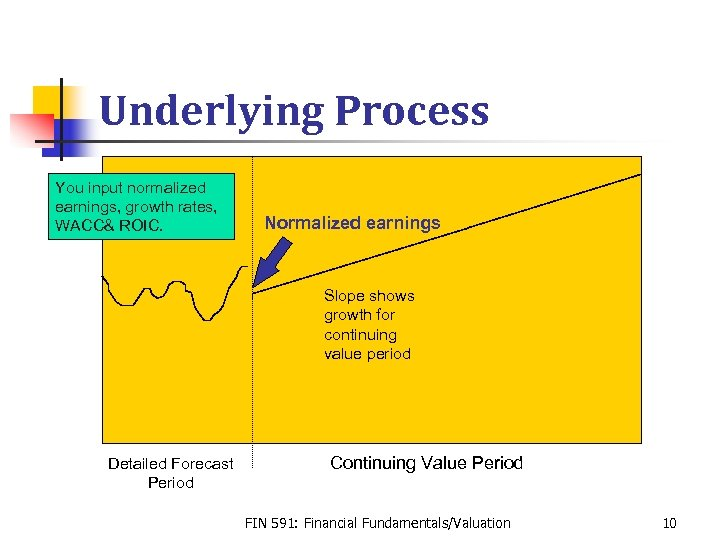Underlying Process You input normalized earnings, growth rates, WACC& ROIC. Normalized earnings Slope shows growth for continuing value period Detailed Forecast Period Continuing Value Period FIN 591: Financial Fundamentals/Valuation 10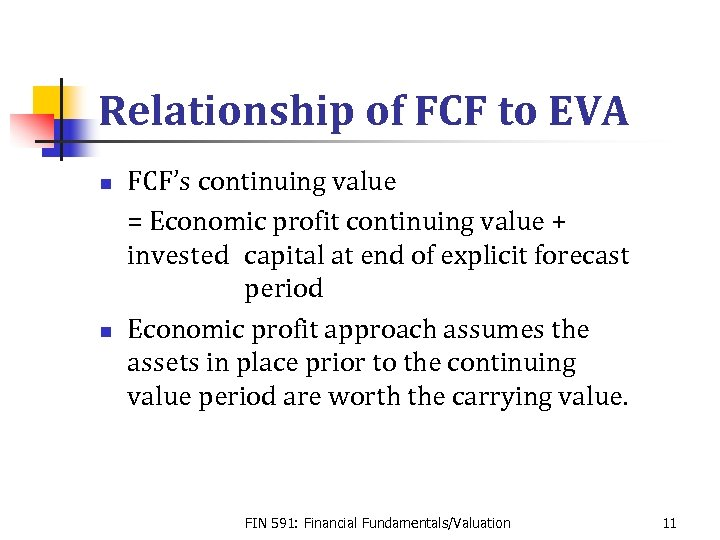Relationship of FCF to EVA n n FCF’s continuing value = Economic profit continuing value + invested capital at end of explicit forecast period Economic profit approach assumes the assets in place prior to the continuing value period are worth the carrying value. FIN 591: Financial Fundamentals/Valuation 11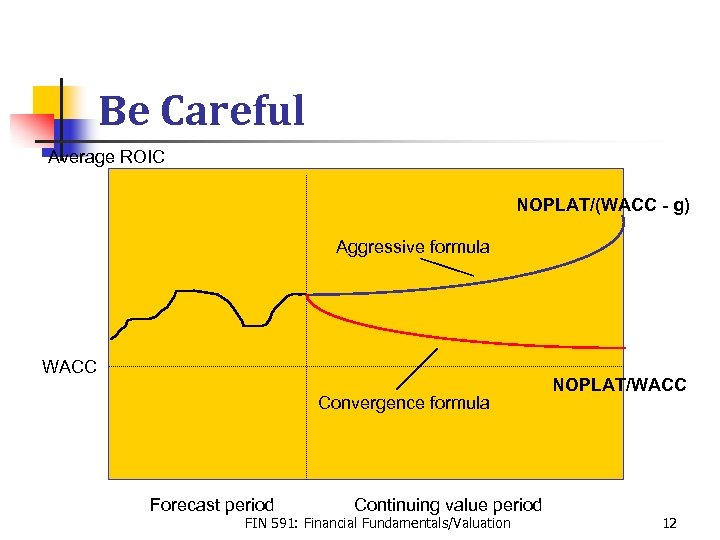Be Careful Average ROIC NOPLAT/(WACC - g) Aggressive formula WACC Convergence formula Forecast period Continuing value period FIN 591: Financial Fundamentals/Valuation NOPLAT/WACC 12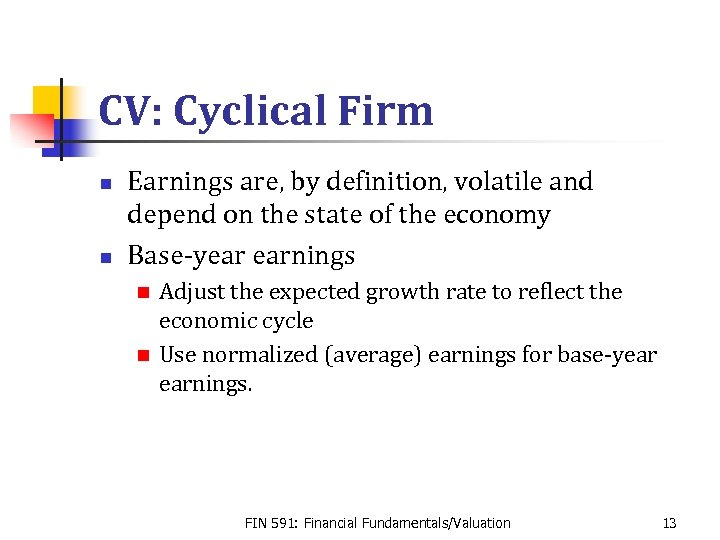CV: Cyclical Firm n n Earnings are, by definition, volatile and depend on the state of the economy Base-year earnings Adjust the expected growth rate to reflect the economic cycle n Use normalized (average) earnings for base-year earnings. n FIN 591: Financial Fundamentals/Valuation 13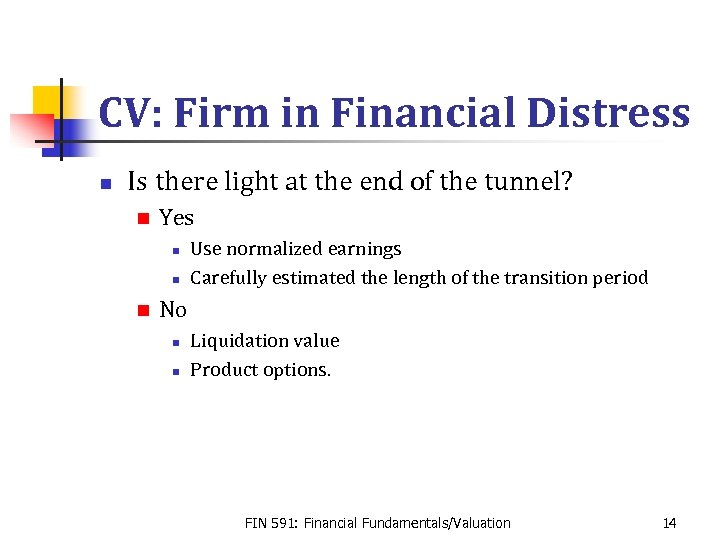CV: Firm in Financial Distress n Is there light at the end of the tunnel? n Yes n n n Use normalized earnings Carefully estimated the length of the transition period No n n Liquidation value Product options. FIN 591: Financial Fundamentals/Valuation 14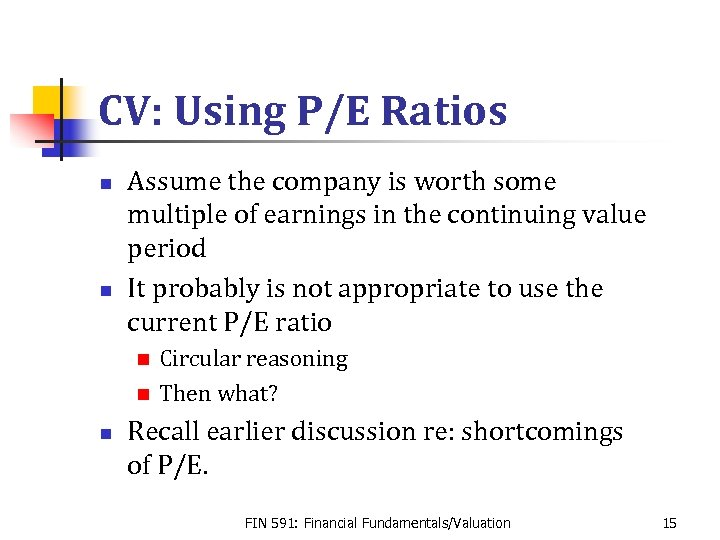CV: Using P/E Ratios n n Assume the company is worth some multiple of earnings in the continuing value period It probably is not appropriate to use the current P/E ratio Circular reasoning n Then what? n n Recall earlier discussion re: shortcomings of P/E. FIN 591: Financial Fundamentals/Valuation 15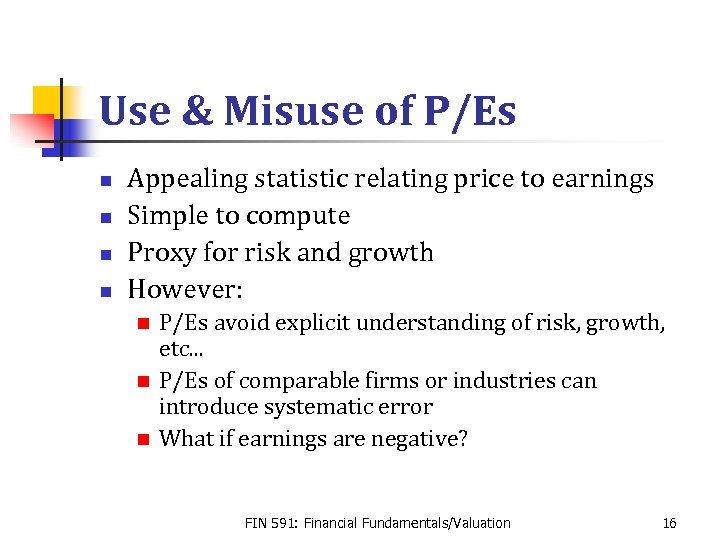Use & Misuse of P/Es n n Appealing statistic relating price to earnings Simple to compute Proxy for risk and growth However: P/Es avoid explicit understanding of risk, growth, etc. . . n P/Es of comparable firms or industries can introduce systematic error n What if earnings are negative? n FIN 591: Financial Fundamentals/Valuation 16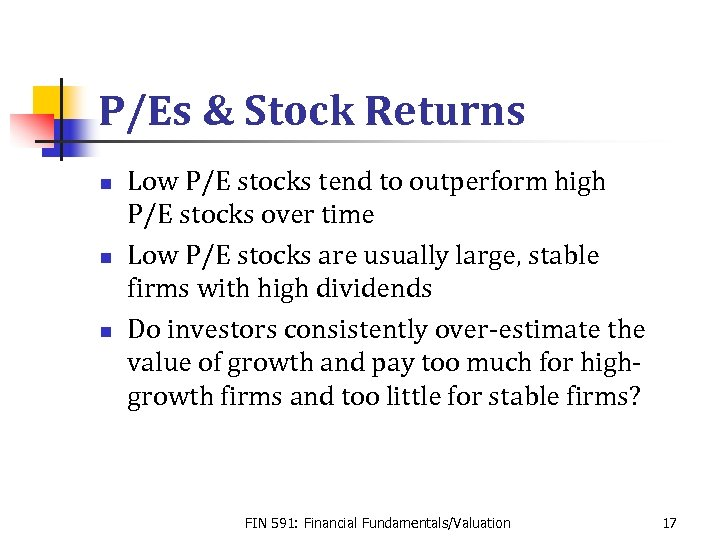P/Es & Stock Returns n n n Low P/E stocks tend to outperform high P/E stocks over time Low P/E stocks are usually large, stable firms with high dividends Do investors consistently over-estimate the value of growth and pay too much for highgrowth firms and too little for stable firms? FIN 591: Financial Fundamentals/Valuation 17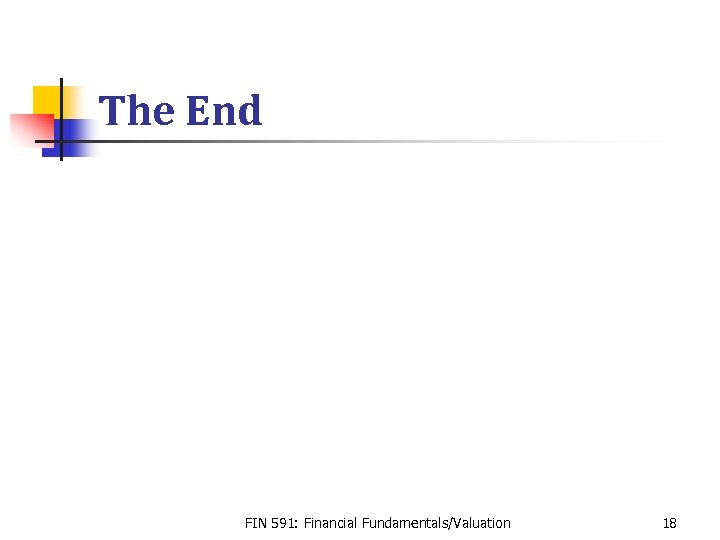The End FIN 591: Financial Fundamentals/Valuation 18